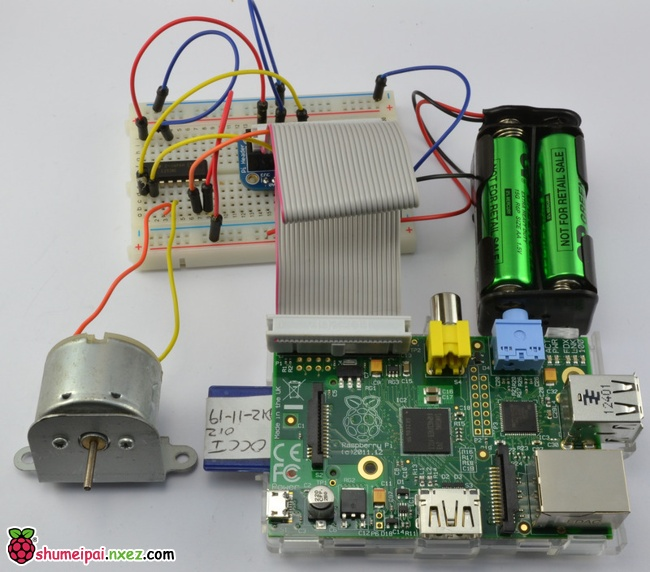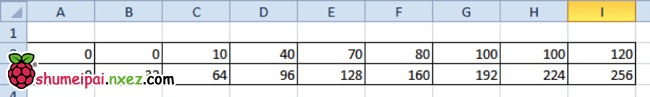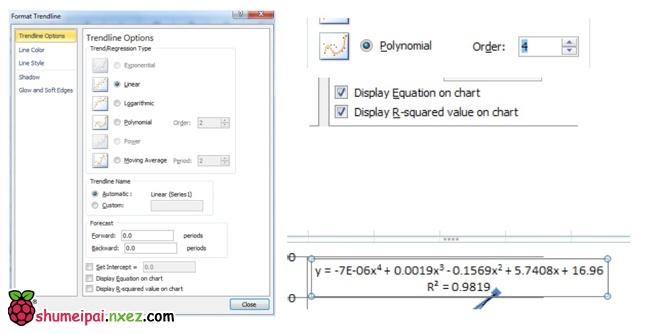# 用逆向设计和回归算法估算PWMy = -7E-06×4 + 0.0019×3 – 0.1569×2 + 5.7408x + 16.96 写进程序里就是：

```int x = target_speed;//需要的速度
int pwm = int(-0.000007 * power(x,4) + 0.0019 * power(x, 3) - 0.1569 * power(x,2) + 5.7408 * x + 16.96);//根据EXCEL给的公式把速度换算成PWM。
```